Scalar product - Defination

Chapter 10 Class 12 Vector Algebra
Concept wise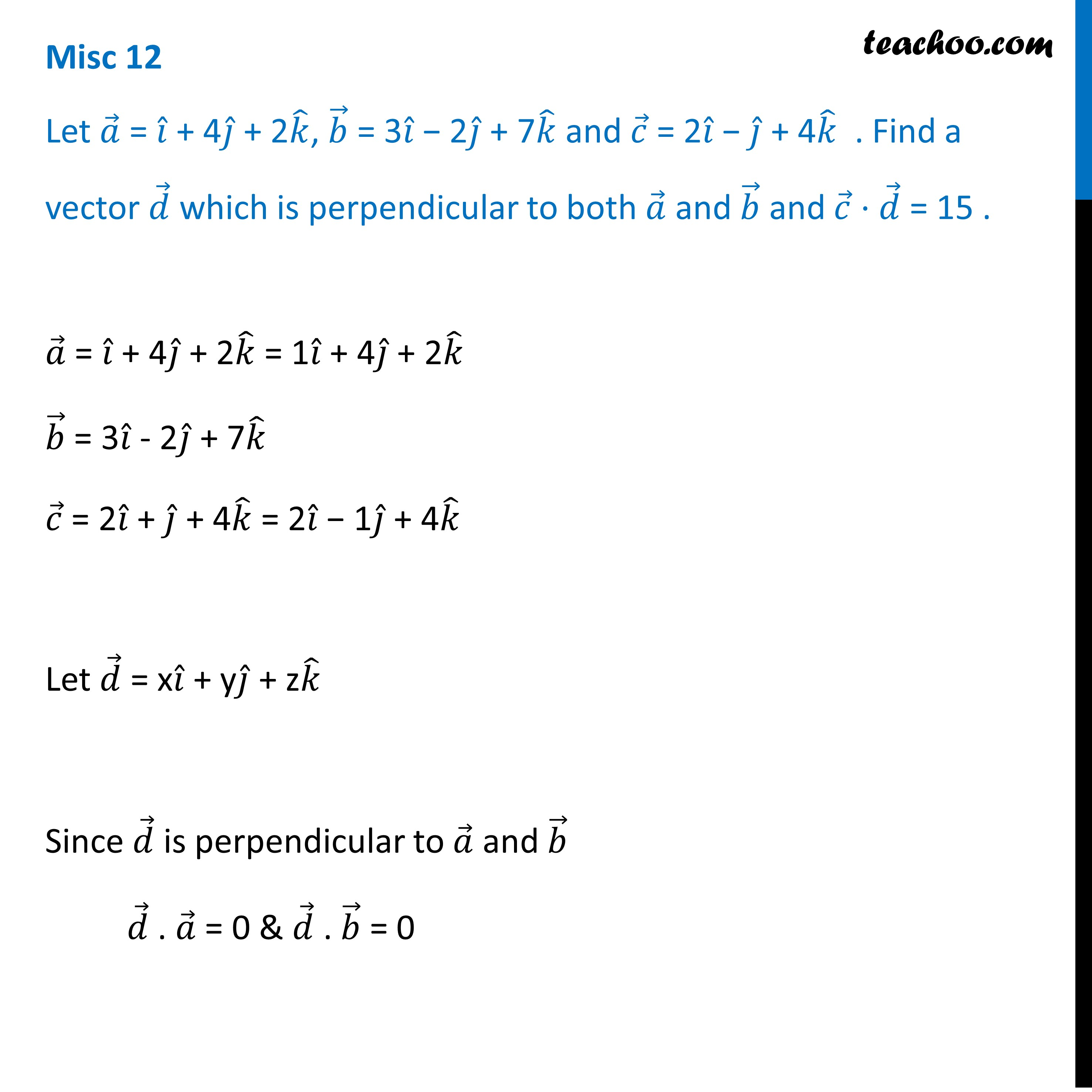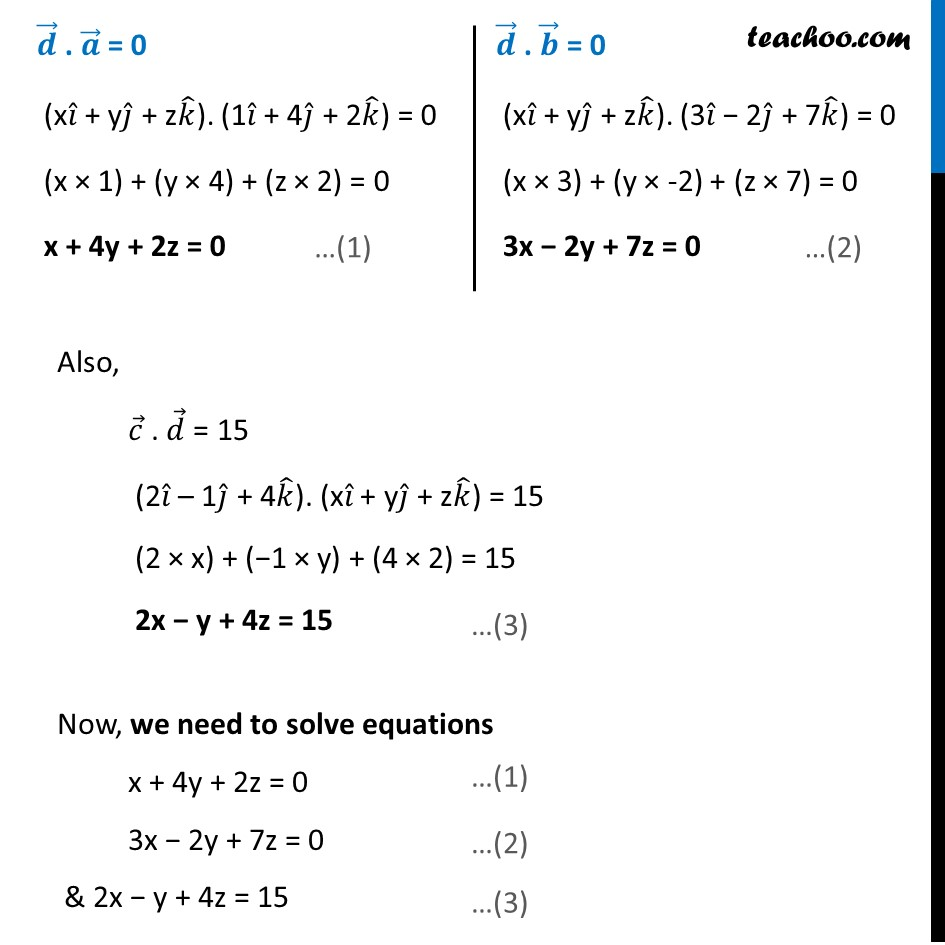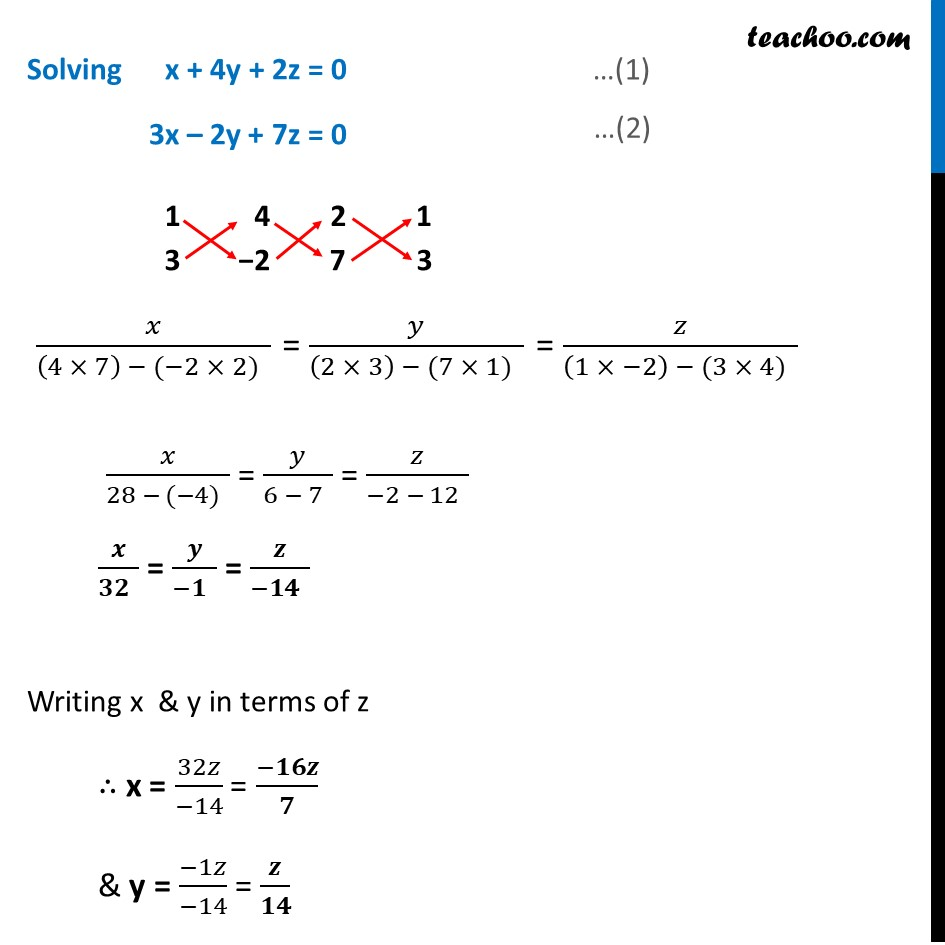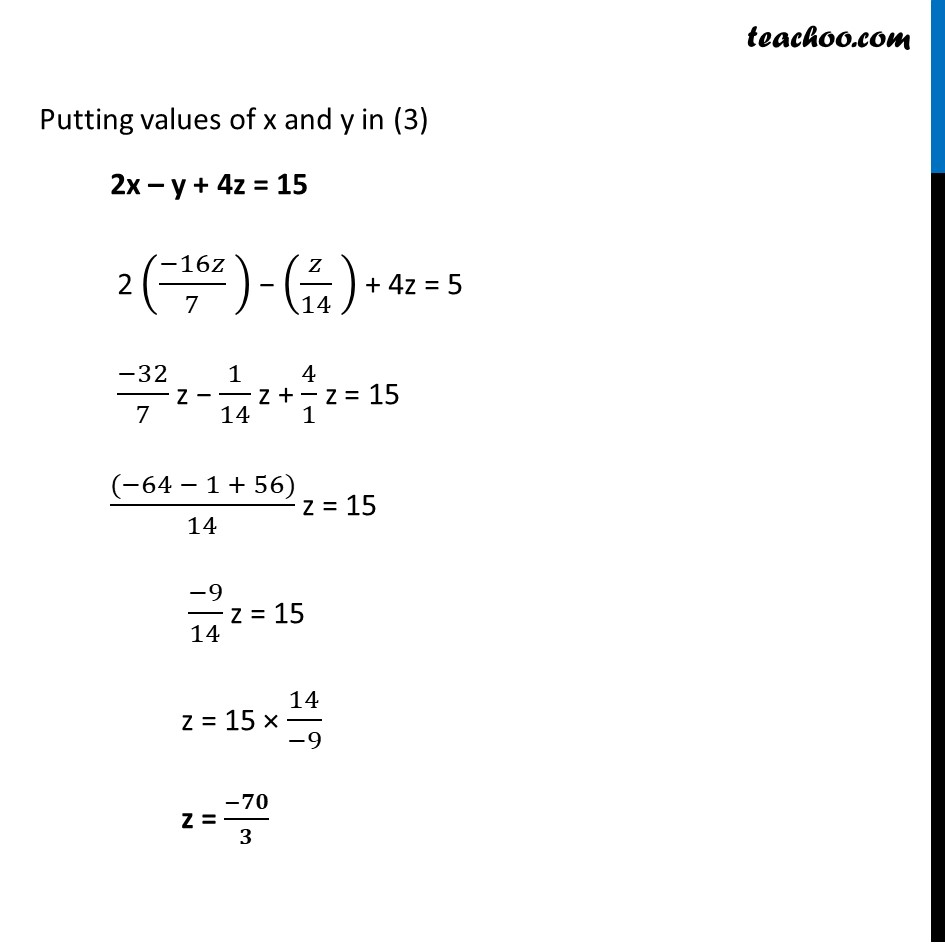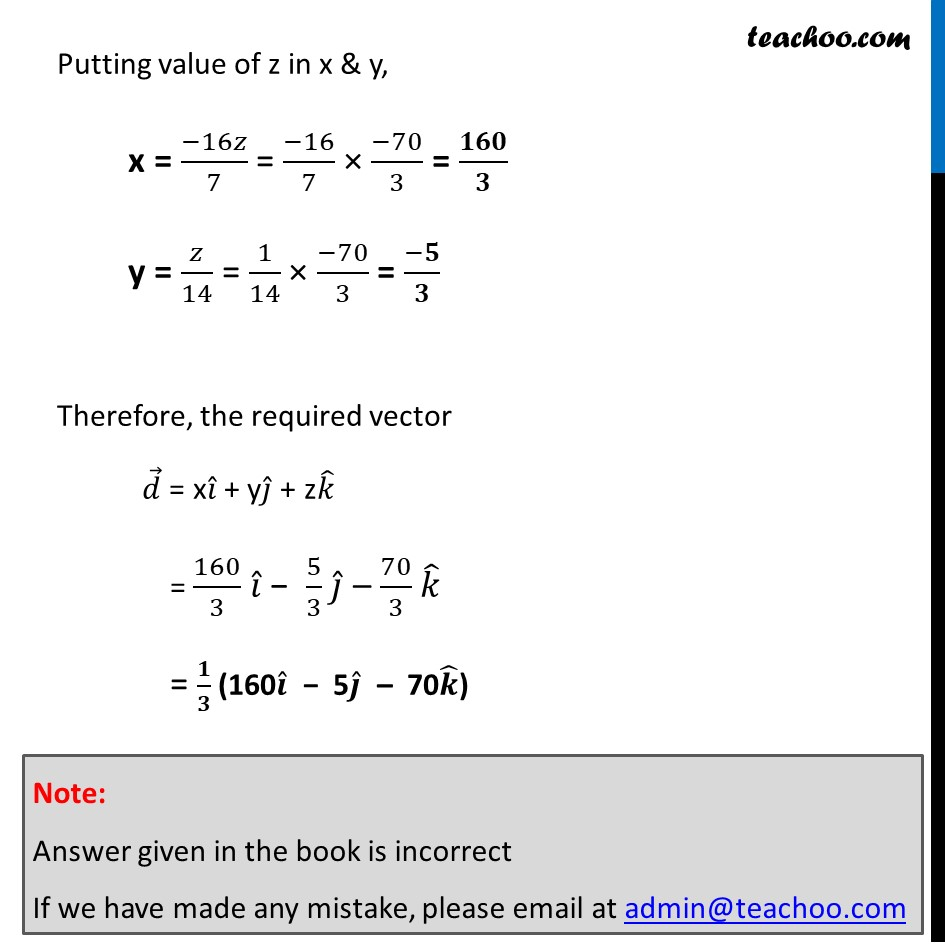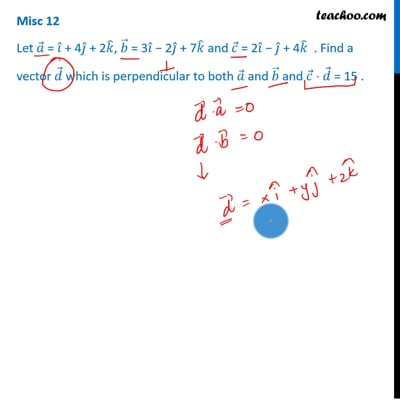This video is only available for Teachoo black users

Introducing your new favourite teacher - Teachoo Black, at only ₹83 per month

### Transcript

Misc 12 Let 𝑎 ⃗ = 𝑖 ̂ + 4𝑗 ̂ + 2𝑘 ̂, 𝑏 ⃗ = 3𝑖 ̂ − 2𝑗 ̂ + 7𝑘 ̂ and 𝑐 ⃗ = 2𝑖 ̂ − 𝑗 ̂ + 4𝑘 ̂ . Find a vector 𝑑 ⃗ which is perpendicular to both 𝑎 ⃗ and 𝑏 ⃗ and 𝑐 ⃗ ⋅ 𝑑 ⃗ = 15 . Given 𝒂 ⃗ = 𝑖 ̂ + 4𝑗 ̂ + 2𝑘 ̂ 𝒃 ⃗ = 3𝑖 ̂ - 2𝑗 ̂ + 7𝑘 ̂ 𝒄 ⃗ = 2𝑖 ̂ + 𝑗 ̂ + 4𝑘 ̂ Let 𝒅 ⃗ = x𝒊 ̂ + y𝒋 ̂ + z𝒌 ̂ Since 𝒅 ⃗ is perpendicular to 𝑎 ⃗ and 𝑏 ⃗ 𝑑 ⃗ . 𝑎 ⃗ = 0 & 𝑑 ⃗ . 𝑏 ⃗ = 0 𝒅 ⃗ . 𝒂 ⃗ = 0 (x𝑖 ̂ + y𝑗 ̂ + z𝑘 ̂). (1𝑖 ̂ + 4𝑗 ̂ + 2𝑘 ̂) = 0 (x × 1) + (y × 4) + (z × 2) = 0 x + 4y + 2z = 0 𝒅 ⃗ . 𝒃 ⃗ = 0 (x𝑖 ̂ + y𝑗 ̂ + z𝑘 ̂). (3𝑖 ̂ − 2𝑗 ̂ + 7𝑘 ̂) = 0 (x × 3) + (y × -2) + (z × 7) = 0 3x − 2y + 7z = 0 Also, 𝑐 ⃗ . 𝑑 ⃗ = 15 (2𝑖 ̂ – 1𝑗 ̂ + 4𝑘 ̂). (x𝑖 ̂ + y𝑗 ̂ + z𝑘 ̂) = 15 (2 × x) + (−1 × y) + (4 × 2) = 15 2x − y + 4z = 15 Now, we need to solve equations x + 4y + 2z = 0 3x − 2y + 7z = 0 & 2x − y + 4z = 15 Solving x + 4y + 2z = 0 3x – 2y + 7z = 0 𝑥/(28 − (−4) ) = 𝑦/(6 − 7 ) = 𝑧/(−2 − 12 ) 𝒙/(𝟑𝟐 ) = 𝒚/(−𝟏 ) = 𝒛/(−𝟏𝟒 ) Writing x & y in terms of z ∴ x = 32𝑧/(−14) = (−𝟏𝟔𝒛)/𝟕 & y = (−1𝑧)/(−14) = 𝒛/𝟏𝟒 Putting values of x and y in (3) 2x – y + 4z = 15 2 ((−16𝑧)/7 ) − (𝑧/14 ) + 4z = 5 (−32)/7 z − 1/14 z + 4/1 z = 15 ((−64 − 1 + 56))/14 z = 15 (−9)/14 z = 15 z = 15 × 14/(−9) z = (−𝟕𝟎)/𝟑 Putting value of z in x & y, x = (−16𝑧)/7 = (−16)/7 × (−70)/3 = 𝟏𝟔𝟎/𝟑 y = 𝑧/14 = 1/14 × (−70)/3 = (−𝟓)/𝟑 Therefore, the required vector 𝑑 ⃗ = x𝑖 ̂ + y𝑗 ̂ + z𝑘 ̂ = 160/3 𝑖 ̂ − 5/3 𝑗 ̂ – 70/3 𝑘 ̂ = 𝟏/𝟑 (160𝒊 ̂ − 5𝒋 ̂ – 70𝒌 ̂) Note: Answer given in the book is incorrect If we have made any mistake, please email at admin@teachoo.com